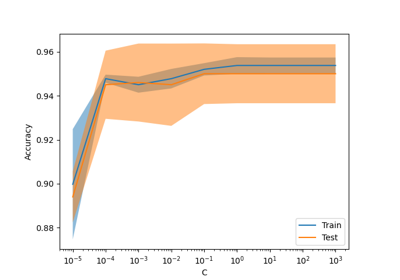# sklearn.datasets.make_low_rank_matrix¶

sklearn.datasets.make_low_rank_matrix(n_samples=100, n_features=100, *, effective_rank=10, tail_strength=0.5, random_state=None)[source]

Generate a mostly low rank matrix with bell-shaped singular values.

Most of the variance can be explained by a bell-shaped curve of width effective_rank: the low rank part of the singular values profile is:

(1 - tail_strength) * exp(-1.0 * (i / effective_rank) ** 2)


The remaining singular values’ tail is fat, decreasing as:

tail_strength * exp(-0.1 * i / effective_rank).


The low rank part of the profile can be considered the structured signal part of the data while the tail can be considered the noisy part of the data that cannot be summarized by a low number of linear components (singular vectors).

This kind of singular profiles is often seen in practice, for instance:
• gray level pictures of faces

• TF-IDF vectors of text documents crawled from the web

Read more in the User Guide.

Parameters:
n_samplesint, default=100

The number of samples.

n_featuresint, default=100

The number of features.

effective_rankint, default=10

The approximate number of singular vectors required to explain most of the data by linear combinations.

tail_strengthfloat, default=0.5

The relative importance of the fat noisy tail of the singular values profile. The value should be between 0 and 1.

random_stateint, RandomState instance or None, default=None

Determines random number generation for dataset creation. Pass an int for reproducible output across multiple function calls. See Glossary.

Returns:
Xndarray of shape (n_samples, n_features)

The matrix.

## Examples using sklearn.datasets.make_low_rank_matrix¶Release Highlights for scikit-learn 1.3

Release Highlights for scikit-learn 1.3